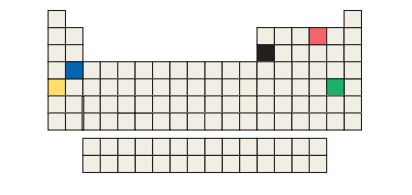×
Get Full Access to Chemistry: The Central Science - 14 Edition - Chapter 2 - Problem 2.7
Get Full Access to Chemistry: The Central Science - 14 Edition - Chapter 2 - Problem 2.7

×

# ?Five of the boxes in the following periodic table are colored. Predict the charge on the ion associated with each of these elements. [Section 2.7]ISBN: 9780134414232 1274

## Solution for problem 2.7 Chapter 2

Chemistry: The Central Science | 14th Edition

• Textbook Solutions
• 2901 Step-by-step solutions solved by professors and subject experts
• Get 24/7 help from StudySoup virtual teaching assistantsChemistry: The Central Science | 14th Edition

4 5 1 299 Reviews
19
2
Problem 2.7

Five of the boxes in the following periodic table are colored. Predict the charge on the ion associated with each of these elements. [Section 2.7]Step-by-Step Solution:

Step 1 of 5) Five of the boxes in the following periodic table are colored. Predict the charge on the ion associated with each of these elements.If electrons are removed from or added to an atom, a charged particle called an ion is formed. An ion with a positive charge is a cation (pronounced CAT-ion); a negatively charged ion is an anion (AN-ion). To see how ions form, consider the sodium atom, which has 11 protons and 11 electrons. This atom easily loses one electron. The resulting cation has 11 protons and 10 electrons, which means it has a net charge of 1+.The net charge on an ion is represented by a superscript. The superscripts +, 2+, and 3+, for instance, mean a net charge resulting from the loss of one, two, and three electrons, respectively. The superscripts -, 2-, and 3- represent net charges resulting from the gain of one, two, and three electrons, respectively. Chlorine, with 17 protons and 17 electrons, for example, can gain an electron in chemical reactions, producing the Cl - ion:

Step 2 of 2

##### ISBN: 9780134414232

The full step-by-step solution to problem: 2.7 from chapter: 2 was answered by , our top Chemistry solution expert on 10/03/18, 06:29PM. Since the solution to 2.7 from 2 chapter was answered, more than 215 students have viewed the full step-by-step answer. The answer to “?Five of the boxes in the following periodic table are colored. Predict the charge on the ion associated with each of these elements. [Section 2.7]” is broken down into a number of easy to follow steps, and 25 words. This textbook survival guide was created for the textbook: Chemistry: The Central Science, edition: 14. Chemistry: The Central Science was written by and is associated to the ISBN: 9780134414232. This full solution covers the following key subjects: . This expansive textbook survival guide covers 29 chapters, and 2820 solutions.

## Discover and learn what students are asking

Calculus: Early Transcendental Functions : Area and Arc Length in Polar Coordinates
?In Exercises 5-16, find the area of the region. Interior of $$r=3 \cos \theta$$

Calculus: Early Transcendental Functions : Conservative Vector Fields and Independence of Path
?In Exercises 1 - 4, show that the value of $$\int_{C} F \cdot d r$$ is the same for each parametric representation of C. \(\mathbf{F}(x, y)=y

Statistics: Informed Decisions Using Data : Tests for Independence and the Homogeneity of Proportions
?In a chi-square test for _______ of proportions, we test whether different populations have the same proportion of individuals with some characteristi

Unlock Textbook Solution